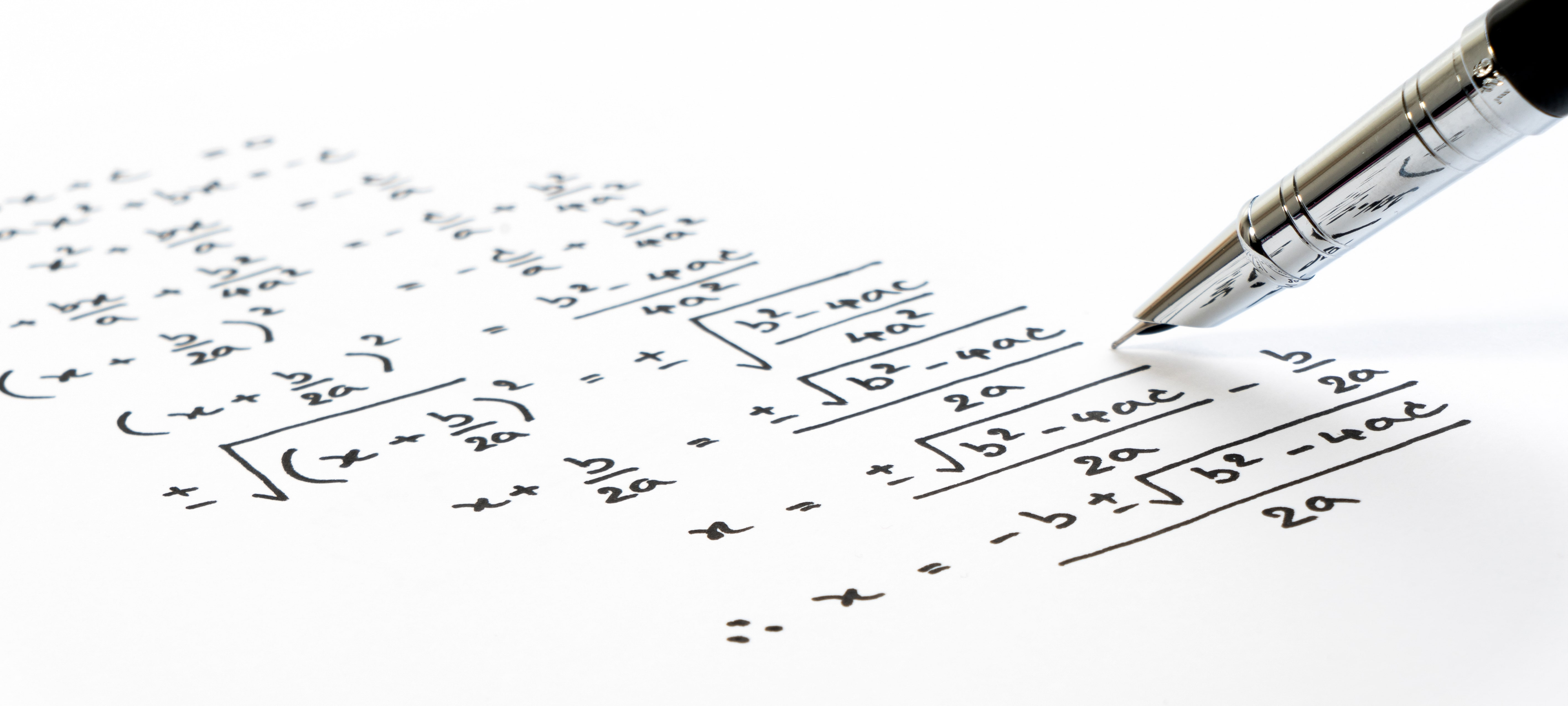# Benefits to Writing Algebra Equations in Standard Form With a Tutor in Torrance

Learning how to graph linear equations is a big part of Algebra, and unfortunately, not all tests will allow your student help from a graphing calculator. Good news, an A Plus In Home Algebra Tutor in Torrance can teach you super simple graphing tips that will take away your linear equation headaches!# Benefits to Writing Algebra Equations in Standard Form With a Tutor in Torrance

Last Updated on September 30, 2020 by A Plus In-Home Tutors

Learning how to graph linear equations is a big part of Algebra, and unfortunately, not all tests will allow your student help from a graphing calculator.

# Good news, an A Plus In Home Algebra Tutor in Torrance can teach you super simple graphing tips that will take away your linear equation headaches!

## In Algebra, slope intercept form is one way to graph linear equations, but there’s another, often easier way – convert the Algebra equation to standard form and find the x and y intercepts.

Standard form must be written as Ax + By = C, where A and B are coefficients and C is the constant.
In order to figure out how to find the x and y intercepts, let’s first review what an intercept is in Algebra: an intercept is where your line crosses an axis. The point where the line touches the x axis is called the x intercept, and the point where the line touches the y axis is called the y intercept.

Once Algebra equations are written in standard form, it’s easy to find the intercepts. To find the X intercept, let y = 0. To find the Y intercept, you let x = 0. Then, right when we find the points where the line crosses the x and y axis, we’re already able to draw a line!
Here’s an Algebra example:
4x+8y = 16
Find the x intercept
Let y = 0
4x+8(0) = 16
4x = 16
x = 4
X Intercept = (4,0)
Find the y intercept
Let x = 0
4(0)+8y = 16
8y = 16
y = 2
Y intercept = (0,2)
That’s all you need to be able to draw your graph!

So how do you convert an Algebra equation to standard form?
We need the variables x and y to be on the left, and the constant to be on the right. From there, we move terms around like we would with any Algebra equation – whatever you do to one side, make sure you do it to the other!
Say you are given the following Algebra equation:
y = -1/2x – 8
That might be a bit difficult to graph as is (slope intercept form), so make things easier. Let’s convert it to standard form.
Multiply both sides by 2 and you get 2y = -x – 16.
Then, add x to both sides to get:
x + 2y = -16.

### That’s the Algebra equation in standard form!

Let x = 0 and you get y = -8, making the y intercept (0, -8).
Let y = 0 and you get x = -16, making the x intercept (-16,0)
From there, you have two points and can immediately draw your line. Algebra problem solved!
Need more help with graphing linear equations or converting a slope intercept form equation to standard form in Torrance?

#### An expert Algebra tutor from A Plus In Home Tutors can teach you useful tricks and tips.

##### Visit us at www.APlusInHomeTutors.com to find a great Algebra tutor near you!

Posted in Algebra